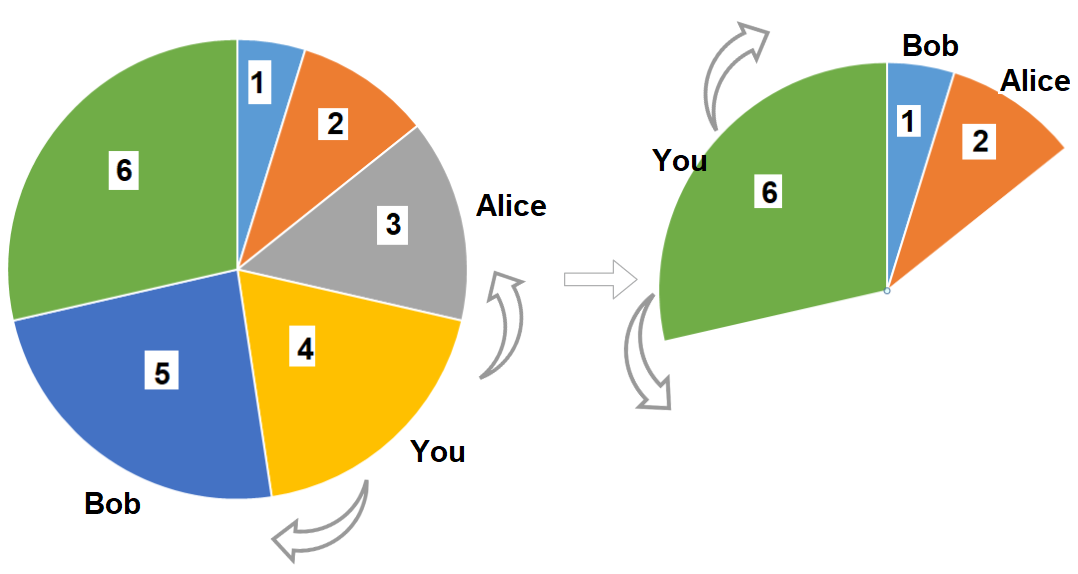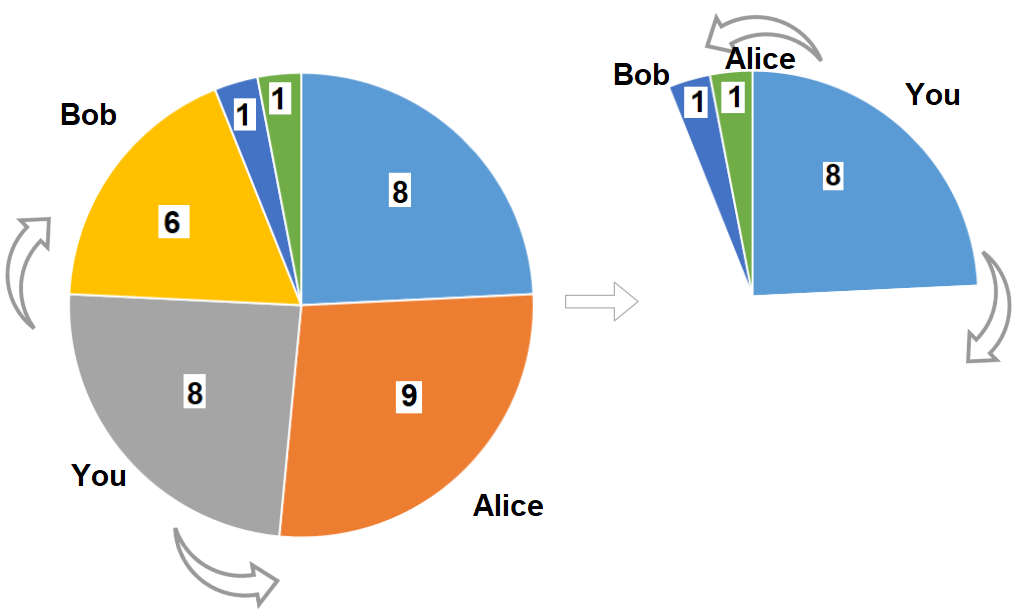# GeetCode Hub

There is a pizza with 3n slices of varying size, you and your friends will take slices of pizza as follows:

• You will pick any pizza slice.
• Your friend Alice will pick next slice in anti clockwise direction of your pick.
• Your friend Bob will pick next slice in clockwise direction of your pick.
• Repeat until there are no more slices of pizzas.

Sizes of Pizza slices is represented by circular array `slices` in clockwise direction.

Return the maximum possible sum of slice sizes which you can have.

Example 1:```Input: slices = [1,2,3,4,5,6]
Output: 10
Explanation: Pick pizza slice of size 4, Alice and Bob will pick slices with size 3 and 5 respectively. Then Pick slices with size 6, finally Alice and Bob will pick slice of size 2 and 1 respectively. Total = 4 + 6.
```

Example 2:```Input: slices = [8,9,8,6,1,1]
Output: 16
Output: Pick pizza slice of size 8 in each turn. If you pick slice with size 9 your partners will pick slices of size 8.
```

Example 3:

```Input: slices = [4,1,2,5,8,3,1,9,7]
Output: 21
```

Example 4:

```Input: slices = [3,1,2]
Output: 3
```

Constraints:

• `1 <= slices.length <= 500`
• `slices.length % 3 == 0`
• `1 <= slices[i] <= 1000`

class Solution { public int maxSizeSlices(int[] slices) { } }